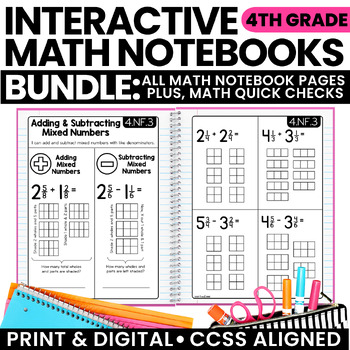# Math Interactive Notebook 4th Grade BUNDLE | Digital and Printable3rd - 5th, Homeschool
Subjects
Standards
Resource Type
Formats Included
• Zip
Pages
220+
\$25.50
Bundle
List Price:
\$31.50
You Save:
\$6.00
\$25.50
Bundle
List Price:
\$31.50
You Save:
\$6.00
##### Also included in
1. This BUNDLE includes everything you need for 4th grade math!!★ Interactive Notebook Pages★ Math Quick Checks ★ Math Menus (Perfect for homework or early finishers!!)★ Open-Ended Math Pages★ Math Mastery Task Cards★ Units for Fractions, Decimals, Measurement & GeometryFeel free to email with any
\$82.50
\$103.70
Save \$21.20

### Description

What are Interactive Math Notebooks?

Interactive math notebooks are teaching pages used to introduce and teach math concepts and skills. Each set of notebook pages includes definitions, explanations, rules, and algorithms that may be needed to address each standard. Practice problems are included to be used for guided practice. Once completed, these pages serve as a great reference tool for students to refer back throughout the year.

How do I use them with my students?

Notebook pages can be used to introduce, reinforce, or review math standards. They can be used for direct instruction for a whole class or in small groups. For the printable version, students first cut out the pages and glue them into a spiral notebook. With teacher guidance, students take notes and work out practice problems. Students can then highlight or add color to their notebook pages. The digital pages include the same content, but require no cutting or pasting!

What materials are needed for printable notebook pages?

A spiral notebook for each student, scissors, glue sticks, pencils, and colored pencils, crayons, markers (optional)

What is included in this BUNDLE?

• Notebook pages for each of the 4th grade math standards. Each concept or skill includes a double-page spread. (Printable & Digital)
• Answer Key (Available with printable pages)
• Math Quick Checks for all 4th grade math standards

★★This resources in this bundle are also included in my ULTIMATE 4th Grade Math Bundle!!★★

(For a more detailed PREVIEW, please preview each of the individual products above!)

★★This resource is included in myULTIMATE 4th Grade Math Bundle!!★★

Check out the rest of my Math Activities for 4th Grade:

4th Grade Interactive Math Notebook: OA

4th Grade Interactive Math Notebook: NBT

4th Grade Interactive Math Notebook: NF

4th Grade Interactive Math Notebook: MD

4th Grade Interactive Math Notebook: G

Common Core Math Quick Checks

Math Exit Slips

Solve & Sort Math Centers

Total Pages
220+
Included
Teaching Duration
1 Year
Report this Resource to TpT
Reported resources will be reviewed by our team. Report this resource to let us know if this resource violates TpT’s content guidelines.

### Standards

to see state-specific standards (only available in the US).
Interpret a multiplication equation as a comparison, e.g., interpret 35 = 5 Ã 7 as a statement that 35 is 5 times as many as 7 and 7 times as many as 5. Represent verbal statements of multiplicative comparisons as multiplication equations.
Multiply or divide to solve word problems involving multiplicative comparison, e.g., by using drawings and equations with a symbol for the unknown number to represent the problem, distinguishing multiplicative comparison from additive comparison.
Solve multistep word problems posed with whole numbers and having whole-number answers using the four operations, including problems in which remainders must be interpreted. Represent these problems using equations with a letter standing for the unknown quantity. Assess the reasonableness of answers using mental computation and estimation strategies including rounding.
Find all factor pairs for a whole number in the range 1-100. Recognize that a whole number is a multiple of each of its factors. Determine whether a given whole number in the range 1-100 is a multiple of a given one-digit number. Determine whether a given whole number in the range 1-100 is prime or composite.
Generate a number or shape pattern that follows a given rule. Identify apparent features of the pattern that were not explicit in the rule itself. For example, given the rule âAdd 3â and the starting number 1, generate terms in the resulting sequence and observe that the terms appear to alternate between odd and even numbers. Explain informally why the numbers will continue to alternate in this way.